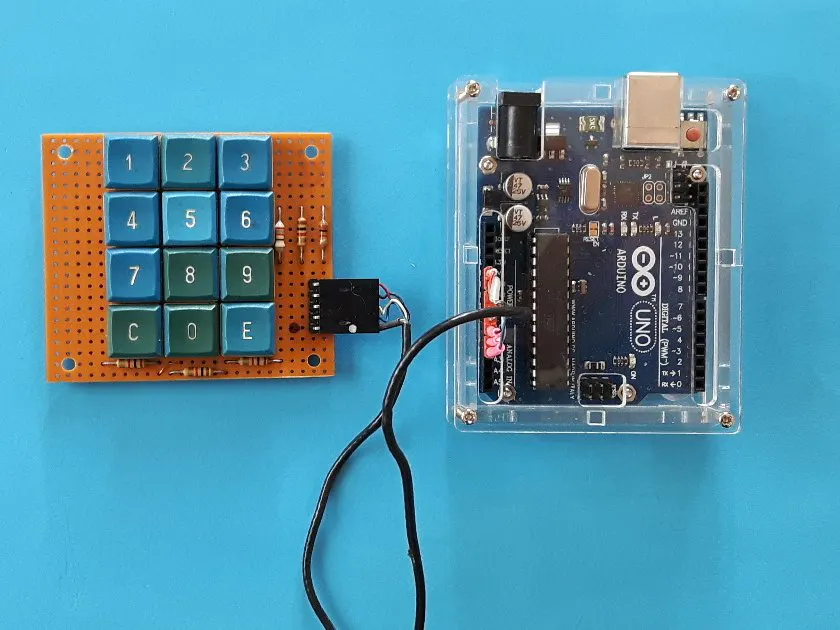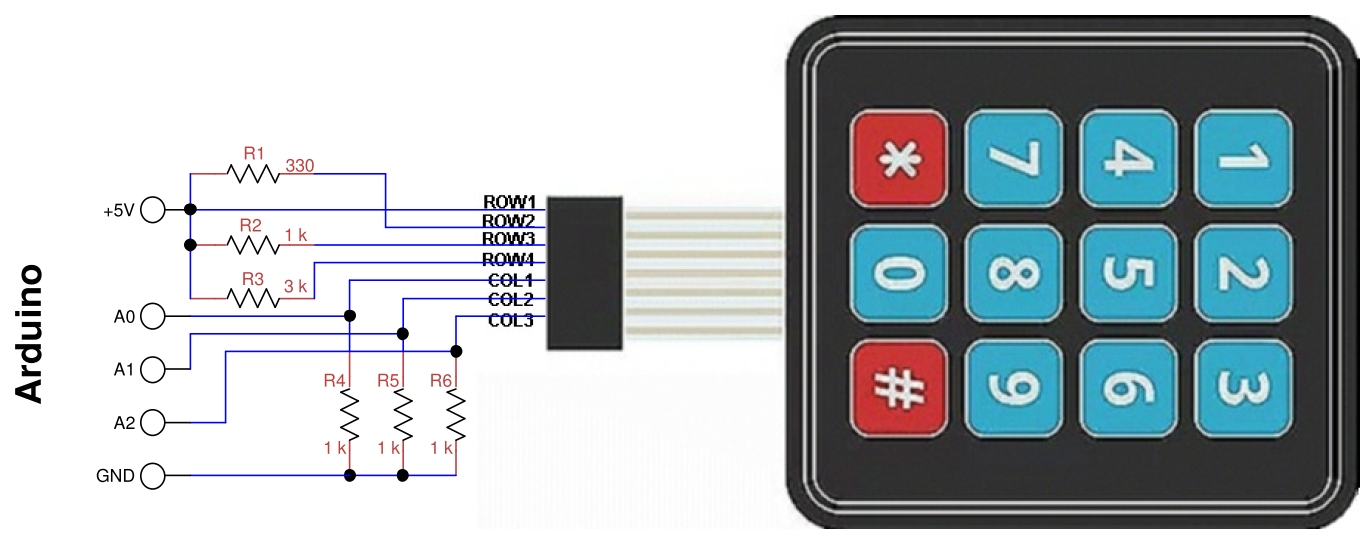Project tutorialA technique for drastically reducing the pins required by a matrix keypad is described.

• 3,817 views
• 14 respects

## Necessary tools and machinesSoldering iron (generic)

## Apps and online services

### Introduction

The aim of this project is to significantly reduce the number of pins required by a numeric keypad. This is because we often need many I/O pins compared to those available on the Arduino Uno or Nanoboards.

The ordinary numerical keypads are structured in matrix: a 4x3 keypad, requires 4+3 Arduino digital pins. Numerous libraries are available on the net to use it with Arduino.

### My Solution

The solution I propose is much better because it uses only three analog inputs, with a circuit like the one shown in thefollowing figure.

For each column there are only 4 voltage values, corresponding to each of the 4 keys, plus zero volts in case no key is pressed. In the equivalent scheme (b) the R1e indicates one of the four resistors R1-R4, while R2e is one of the three resistors R5-R7.

Now let's see how to make the four widest possible intervals. First of all the resistors R2e = R5 = R6 = R7 can be made equal, then we can set R1 = 0 to have the outputs corresponding to the first line at 5V.

With R2=330 ohm, R3= 1 k ohm, R4= 3 k ohm and R5, R6, R7= 1 k ohm, the following intervals are obtained for each output:

As you can see, the intervals on the threeoutputs are the largest possible and you can use six standard resistors with atolerance of ±5%. With an extra analogue input and another resistor, a 4x4keyboard can be used and so on. The following figure shows the connections withArduino.

In the diagram the resistor R1 is connected toline 2 because the one on line 1 has drawn it with a wire, so the resistorreferences have been scaled by one. Pin assignments can be modified accordingto needs, as long as they are pin configurable as analog.

Of course, if Arduino is powered at 3.3V, nothing would change because the ADC converter as default uses the supplyvoltage and the ADC numbers don’t change.

To test the program, not having a keyboardof this type, I built it with recycled keys, the figure below shows myprototype. The 5-pin right connector is used to wire it to Arduino.

## Code

##### Analog4x3Keybf.inoArduino
Simple program to test KeyPad() function
```/* program Analog4x3Keybf
*  test for 4x3 keys keyboard with 3 analog outs
*  G. Carrera - 19/11/2018
*/

// limits of keyboard output values:
const char key = {'1','4','7','C','2','5','8','0','3','6','9','E'};
int keyval;
int i,colp,val;

void setup(){
Serial.begin(9600); // used with serial monitor
}

void loop() {
char k;
if (k != 'N'){ // a key was pressed
Serial.print("key = ");
Serial.println(k);
delay(500);
}
}

/******** Functions used by program ********/
// key = 'N' for none
if (keyval+keyval+keyval < 40) {
return 'N';
}
else { // a key was pressed
delay(10);// antibounce
for (i=0; i < 3; i++){//identify which column it belongs to
if (keyval[i] > 40){
colp= i;
val= keyval[i];// this is the corresponding value
for (int j=0; j < 4; j++){// identify which key was pressed on the column
return key[colp*4+j];
break;
}
}
}
}
}
}

delay(1);
delay(1);
delay(1);
}
```

## Schematics#### Arduino Keyless Door Lock System with Keypad and LCD

Project tutorial by DIY Hacking

• 36,225 views
• 61 respects

Project in progress by The Gamers

• 3,436 views
• 16 respects

#### Using 4×4 Keypad With Arduino

• 39,428 views
• 35 respects

Project showcase by thehack904

• 18,402 views
• 56 respects

#### I2C Matrix Keypad With PCF8574/PCF8574A GPIO and Visuino

Project tutorial by Boian Mitov

• 8,477 views